# Statistics for Social Science

Lead Author(s): Stephen Hayward

Student Price: Contact us to learn more

Statistics for Social Science takes a fresh approach to the introductory class. With learning check questions, embedded videos and interactive simulations, students engage in active learning as they read. An emphasis on real-world and academic applications help ground the concepts presented. Designed for students taking an introductory statistics course in psychology, sociology or any other social science discipline.

This content has been used by 8,525 students

## What is a Top Hat Textbook?

Top Hat has reimagined the textbook – one that is designed to improve student readership through interactivity, is updated by a community of collaborating professors with the newest information, and accessed online from anywhere, at anytime.

• Top Hat Textbooks are built full of embedded videos, interactive timelines, charts, graphs, and video lessons from the authors themselves
• High-quality and affordable, at a significant fraction in cost vs traditional publisher textbooks

## Key features in this textbookOur Statistics for Social Science textbook allows students to manipulate data, visualize the effects discussed, and explore Lightboard videos that feature instructor explanations to reinforce concepts and calculations.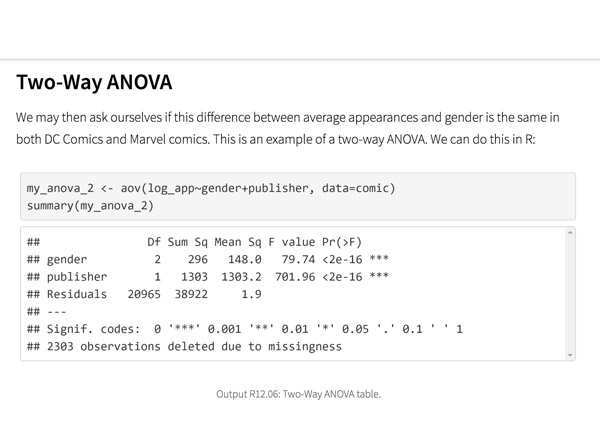Top Hat’s interactive offering includes a complementary module on using R software for data management, graphics, and conducting statistical analyses with examples and practice questions.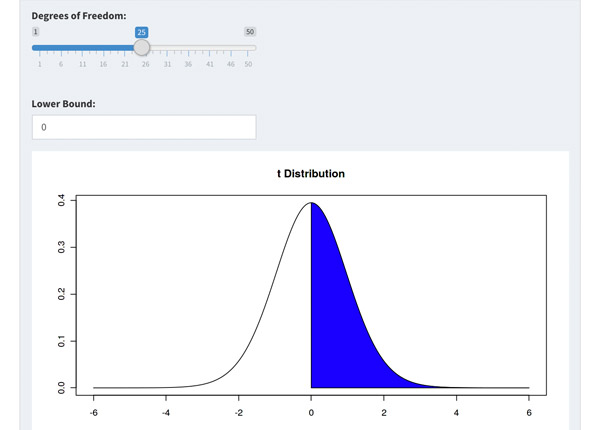Built-in assessment questions embedded throughout chapters so students can read a little, do a little, and test themselves to see what they know!

## Comparison of Social Sciences Textbooks

Consider adding Top Hat’s Statistics for Social Sciences textbook to your upcoming course. We’ve put together a textbook comparison to make it easy for you in your upcoming evaluation.

### Top Hat

Steve Hayward et al., Statistics for Social Sciences, Only one edition needed

### Pearson

Agresti, Statistical Methods for the Social Sciences, 5th Edition

### Cengage

Gravetter et al., Essentials of Statistics for The Behavioral Sciences, 9th Edition

### Sage

Gregory Privitera, Essentials Statistics for the Behavioral Sciences, 2nd Edition

### Pricing

Average price of textbook across most common format

#### Up to40-60%more affordable

Lifetime access on any device

#### $200.83 Hardcover print text only ####$239.95

Hardcover print text only

#### $92 Hardcover print text only ### Always up-to-date content, constantly revised by community of professors Content meets standard for Introduction to Anatomy & Physiology course, and is updated with the latest content ### In-Book Interactivity Includes embedded multi-media files and integrated software to enhance visual presentation of concepts directly in textbook Only available with supplementary resources at additional cost Only available with supplementary resources at additional cost Only available with supplementary resources at additional cost ### Customizable Ability to revise, adjust and adapt content to meet needs of course and instructor ### All-in-one Platform Access to additional questions, test banks, and slides available within one platform ## Pricing Average price of textbook across most common format ### Top Hat Steve Hayward et al., Statistics for Social Sciences, Only one edition needed #### Up to40-60%more affordable Lifetime access on any device ### Pearson Agresti, Statistical Methods for the Social Sciences, 5th Edition ####$200.83

Hardcover print text only

### Pearson

Gravetter et al., Essentials of Statistics for The Behavioral Sciences, 9th Edition

#### $239.95 Hardcover print text only ### Sage McConnell, Brue, Flynn, Principles of Microeconomics, 7th Edition ####$92

Hardcover print text only

## Always up-to-date content, constantly revised by community of professors

Constantly revised and updated by a community of professors with the latest content

### Top Hat

Steve Hayward et al., Statistics for Social Sciences, Only one edition needed

### Pearson

Agresti, Statistical Methods for the Social Sciences, 5th Edition

### Pearson

Gravetter et al., Essentials of Statistics for The Behavioral Sciences, 9th Edition

### Sage

Gregory Privitera, Essentials Statistics for the Behavioral Sciences, 2nd Edition

## In-book Interactivity

Includes embedded multi-media files and integrated software to enhance visual presentation of concepts directly in textbook

### Top Hat

Steve Hayward et al., Statistics for Social Sciences, Only one edition needed

### Pearson

Agresti, Statistical Methods for the Social Sciences, 5th Edition

### Pearson

Gravetter et al., Essentials of Statistics for The Behavioral Sciences, 9th Edition

### Sage

Gregory Privitera, Essentials Statistics for the Behavioral Sciences, 2nd Edition

## Customizable

Ability to revise, adjust and adapt content to meet needs of course and instructor

### Top Hat

Steve Hayward et al., Statistics for Social Sciences, Only one edition needed

### Pearson

Agresti, Statistical Methods for the Social Sciences, 5th Edition

### Pearson

Gravetter et al., Essentials of Statistics for The Behavioral Sciences, 9th Edition

### Sage

Gregory Privitera, Essentials Statistics for the Behavioral Sciences, 2nd Edition

## All-in-one Platform

Access to additional questions, test banks, and slides available within one platform

### Top Hat

Steve Hayward et al., Statistics for Social Sciences, Only one edition needed

### Pearson

Agresti, Statistical Methods for the Social Sciences, 5th Edition

### Pearson

Gravetter et al., Essentials of Statistics for The Behavioral Sciences, 9th Edition

### Sage

Gregory Privitera, Essentials Statistics for the Behavioral Sciences, 2nd Edition

## About this textbook

### Lead Authors

#### Steve HaywardRio Salado College

A lifelong learner, Steve focused on statistics and research methodology during his graduate training at the University of New Mexico. He later founded and served as CEO of Center for Performance Technology, providing instructional design and training development support to larger client organizations throughout the United States. Steve is presently lead faculty member for statistics at Rio Salado College in Tempe, Arizona.

#### Joseph F. Crivello, PhDUniversity of Connecticut

Joseph Crivello has taught Anatomy & Physiology for over 34 years, and is currently a Teaching Fellow and Premedical Advisor of the HMMI/Hemsley Summer Teaching Institute.

## Explore this textbook

Read the fully unlocked textbook below, and if you’re interested in learning more, get in touch to see how you can use this textbook in your course today.

# Descriptive StatisticsThe symmetrical distribution of a snowflake sculpture, taken in Ankara, Turkey. 

## Chapter Objectives

After completing this chapter, you will be able to:

•  Construct a frequency table.
•  Construct charts and graphs to summarize and present data.
•  Find the mean, median, and mode of a population and a sample.
•  Describe a distribution of data in statistical terms.
•  Find fractiles of a data set.
•  Find the variance and standard deviation of a population and a sample.
•  Interpret the variance and standard deviation.

## Summarizing Data

Learning statistics is also about learning to organize and summarize information in a concise and relevant manner. When presented with a seemingly chaotic jumble of numbers, we naturally try to impose some order so as to grasp the information hidden away in them. Much of what you will learn in this course is about techniques to extract information from groups of numbers that comprise the raw data of statistics and present that information in summary form.

Just starting out, one might wonder if the statistician’s role is just to build data tables and graphics, but that is just a starting point, as the characters in the following video point out.

Tables and graphs can be used to summarize sets of numbers, and are a first step in the process of extracting the information they contain. In this chapter, you’ll see not only various ways data can be organized and presented but how it can be summarized in terms of measures that can be calculated.

## Frequency Tables

A frequency table, in its simplest form, is a table showing discrete data values, or scores, together with the frequency of each score. Often, the percentage of the total frequency of each score is displayed in a separate column. Frequency tables are especially useful in that they organize and summarize the raw data in a way that shows the spread, or dispersion, of the data.

Example: Frequency Table

Cups of coffee consumed by ten participants in a morning study group at the Student Union were counted. The results were: 3, 2, 1, 6, 2, 2, 4, 1, 2, 3. Examining the table, we can immediately see how the values, or scores, are dispersed around the highest frequency of 4, the frequency associated with 2 cups of coffee.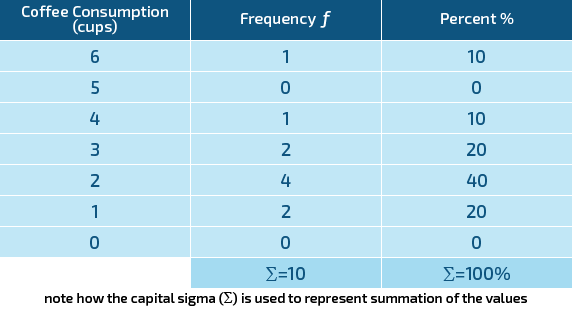Figure 2.1: Coffee consumption​

Examining the table, we can immediately see how the values are dispersed around 4, the highest frequency and the one associated with a value of 2 cups.

Don't think measuring data can save lives? Check out this video for an interesting example of the power of statistics:

## Grouped Frequency Tables

A grouped frequency table is useful when the number of scores is so large that displaying them incrementally would make the table too large to be useful as a summary. The grouped table differs from the previous example of a frequency table in that the data are grouped into classes. Given a large range of values, this enables the data to be summarized more concisely. The trick here is to select an interval that reduces the clutter but doesn’t lose too much information in the process. A good guideline is to use class intervals that are round numbers and that will provide from 5 to 10 intervals, but also are intuitively easy to grasp. Obviously, smaller data sets will require fewer classes, while larger data sets will require more classes.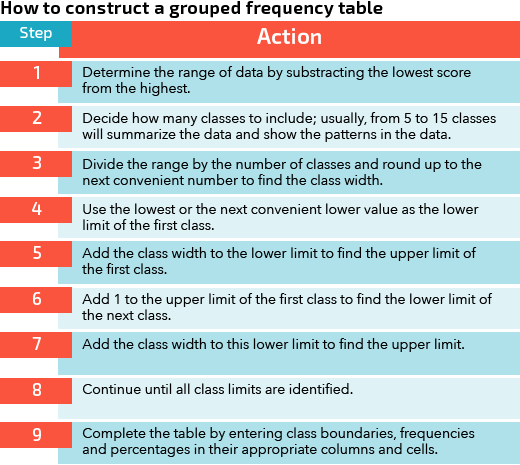Figure 2.2: Constructing a grouped frequency table​

Example: Construct a Grouped Frequency Table​

Here is a hypothetical scenario where constructing a grouped frequency table would be useful. Follow the steps below to practice going through the process of constructing the table.

An instructor recorded placement test scores for 25 students registering for her statistics class. The results were:

12, 72, 26, 77, 38, 34, 55, 67, 64, 51, 43, 48, 69, 77, 73, 44, 32, 8, 36, 78, 74, 33, 77, 72, 32

Arranging the scores in order from lowest to highest, the scores appear as:

8, 12, 26, 32, 32, 33, 34, 36, 38, 43, 44, 48, 51, 55, 64, 67, 69, 72, 72, 73, 74, 77, 77, 77, 78

Just scanning the raw score data is not very informative, as you can see, so the instructor decided to display the data in a frequency table. She proceeded as in the steps below. You can follow along with the steps by viewing the table just below the explanation of the steps.

Step 1: Determine the range of the data by subtracting the lowest score from the highest.

•  The range of the data can be calculated as (highest score) – (lowest score) = (the range of the data).
•  For this data set, the range = 78 – 8 = 70.

Step 2: Decide how many classes to include; usually, anywhere from 5 to 15 classes will summarize the data properly.

•  This can be a matter of judgment and is somewhat arbitrary. Choose a number of classes that will show the spread and general shape of the distribution of scores. With a bit of practice, this will become more intuitive.
•  In this example, the instructor decided to use eight classes to summarize the data.

Step 3: Divide the range by the number of classes and round up to the next convenient number to find the class width.

•  The formula for the class width can be expressed as

Note: It is necessary to always round up to the nearest whole number

•  In this example, the class width = 70/8 = 8.75, rounded up to 9.

Step 4: Use the lowest or the next convenient lower value as the lower limit of the first class.

•  Again, this can be somewhat arbitrary. Choose a lower limit for the first class that will “fit” the data and the number of classes, so that all scores fit within the classes from lowest to highest.
•  Here, the instructor decided to start the lowest class at zero. That enables all of her scores to fit within the eight classes.

Step 5: Add the class width to the lower limit to find the upper limit of the first class.

•  The upper limit of a class can be calculated as (lower limit) + (class width) = (upper limit).
•  The upper limit of the first class was determined to be 0 + 9 = 9.

Step 6: Add 1 to the upper limit of the first class to find the lower limit of the next class.

•  The lower limit of the next class can be calculated as (upper limit of previous class) + 1 = (lower limit of next class).
•  The lower limit of the next class was determined to be 9 + 1 = 10.

Step 7: Add the class width to this lower limit to find the upper limit.

•  The upper limit of the second class was determined to be 10 + 9 = 19.

Step 8: Continue until all class limits are identified.

•  The remaining class boundaries can be determined in like fashion. Stop creating classes once the interval that includes the highest score has been established.

Step 9: Complete the table by entering class boundaries, frequencies, and percentages in their appropriate columns and cells.

•  Enter class boundaries to identify classes in the left column of the table.
•  Enter the number of observations that fall in each class interval in the appropriate row in the column for frequencies. The total of the frequencies should equal the total number of observations. In this example, the frequencies total 25.
•  Determine the percent each frequency is of the total and enter in the percent column. This is also known as the relative frequency.

Calculate relative frequency as

relative frequency = class frequency/ sample size

For the class 70-79, the relative frequency = 8/25 = 32%. The remaining classes can be calculated in like fashion. The percent entries should always total to 100%.Figure 2.3: Placement test scores​

2.01

A ________ table is often used to display data when the data have a very large range of values.

2.02

A grouped frequency table is likely to have between 5 and ________ classes.

2.03

If a grouped frequency table has five classes with the first class 1 – 13, the lower limit of the third class should be ________.

## Charts and Graphs

### Identifying Additional Features of the Data

There are several additional features of data sets that are useful in plotting charts and graphs:

The midpoint of a class is the point midway between the upper and lower class limits. It is calculated by summing the upper and lower limits and dividing that sum by two. You may also see it referred to as the class mark.

The rest of the class midpoints can be determined by simply adding the class width to the class midpoint of the previous class.

The relative frequency of a class is the proportion of the total scores in the data set that are included in that particular class. It may also be expressed as a percentage of the total scores. It is calculated by dividing the class frequency, i.e., the number of scores in that class, by the total number of scores, i.e., the sample size. This is the same calculation that was done in the example above to arrive at frequencies for the grouped table.

The sum of the relative frequencies for all classes should theoretically be equal to 1, or 100%. In practice, the calculated value may vary slightly due to rounding but should nevertheless be very close to those totals.

The cumulative frequency of a class is equal to the sum of the frequency of that class and all classes before that in the distribution. The last class has a cumulative frequency equal to n, i.e., the total sample size.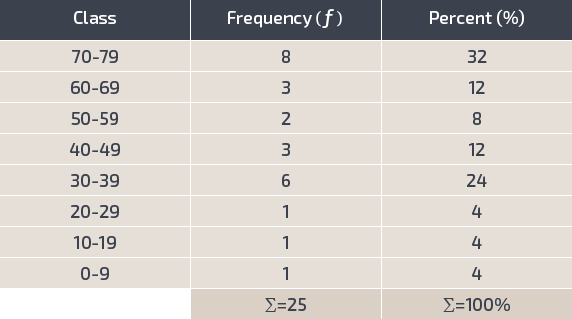Figure 2.3: Placement test scores.
2.04

In the grouped frequency table of placement test scores shown above, the midpoint of the lowest class is $\_\_\_\_\_$.

2.05

The relative frequency of the second class is ______%.

2.06

The cumulative frequency of the last class is ____.

### Frequency Polygons

A frequency polygon can be used to display the same information as a table but provides a different visual take on the data. It is a form of line graph that emphasizes the continuous change in frequencies, thus making it easy to see the “shape” of the distribution and to compare different distributions of data.

To construct the frequency polygon:

Step 1: Construct a frequency table with appropriate classes.

Step 2: Draw the axes of the graph with an x (horizontal) axis and a y (vertical) axis, as in the example here.Figure 2.4: Graph axes labeling​

Step 3: Enter the class limits along the horizontal x-axis.

Step 4: Enter the frequencies along the vertical y-axis.

Step 5: Place dots above the class limits corresponding to the frequency of each class.

Step 6: Connect the dots to form the polygon.

Best practice is to ground the frequency polygon by including a value or class immediately below and above the range of the data so that the polygon begins and ends on the x-axis to close the shape.

Example: Frequency Polygon

Notice how the frequency polygon example below, presenting the same data as the placement test score table above, makes it easy to grasp the spread of the data. It shows clearly how it peaks at one frequency, falls off, then starts to rise again.Figure 2.5: Frequency polygon of placement test scores​

### Histograms and Bar Charts

Histograms and bar charts are another useful way to summarize and present sets of data. The chief difference is that histograms are generally used to show frequencies of numerical data in continuous categories, while bar charts are generally used to show frequencies of categorical data. The difference in presentation can be seen in the examples that follow.

Histogram

To construct a histogram:

Step 1: Construct a frequency table with appropriate classes.

Step 2: Draw the axes of the graph with an x (horizontal) axis and a y (vertical) axis.

Step 3: Enter the class limits along the horizontal x-axis.

Step 4: Enter the frequencies along the vertical y-axis.

Step 5: Draw a bar extending in width from the lower value of each class interval to the lower value of the next interval so that the bars, or columns, are connected with no space between them.

Step 6: Extend those bars to the corresponding frequency level.

Example: Histogram

The histogram example below presents the same data as the placement test score table shown previously. Notice how the same data can be viewed in differing ways by changing the chart type.

Histograms should be used to present continuous numerical data that have been organized into classes. For that reason, the bars are connected to show the continuous nature of the data. Data that are not continuous, or nominal data, are best presented in a bar chart (see the next example, just below the histogram example).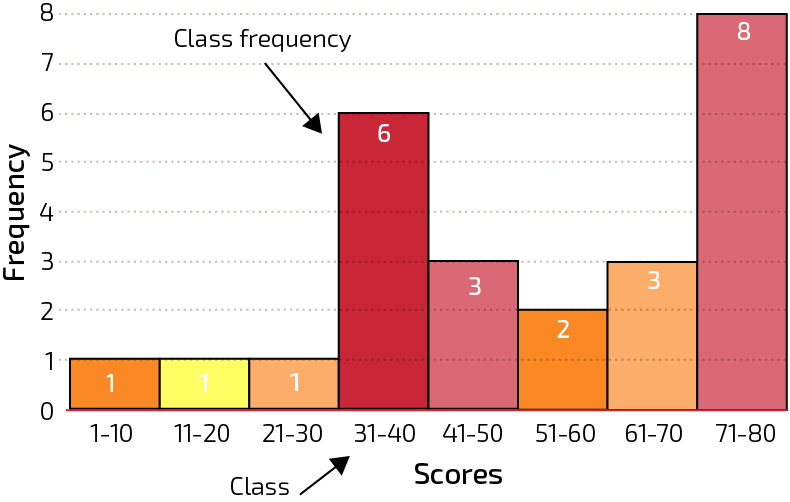Figure 2.6: Histogram of Scores

Bar Chart

A bar chart differs from a histogram in that the columns are not connected. This style of presentation is more appropriate for showing frequencies of nominal categories.

To construct a bar chart:

Step 1: Construct a frequency table with appropriate classes.

Step 2: Draw the axes of the graph with an x (horizontal) axis and a y (vertical) axis.

Step 3: Enter the category descriptions along the horizontal x-axis.

Step 4: Enter the frequencies along the vertical y-axis.

Step 5: Draw a bar centered over the category description.

Step 6: Extend those bars to the corresponding frequency level.

Example: Bar Chart

The bar chart example below presents a hypothetical set of data summarizing the number of student majors among a sample of 150 students at a university.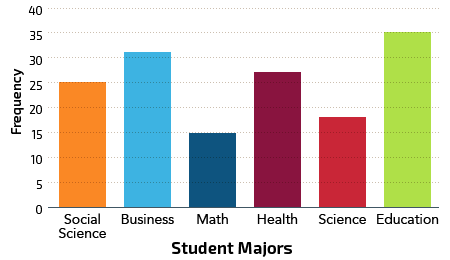Figure 2.7: Bar chart of student majors​

### Pie Charts

A pie chart is a circular graphic that is divided into sectors (commonly referred to as “slices”) to show relative proportions. The advantage it offers is that it makes it easy to visually compare relative frequencies, or proportions, of differing data categories. The area of each sector, or slice, is proportional to the frequency of that category. While a pie chart could be used to show the relative frequency of numerical data classes, they are most often used in conjunction with categorical data.

To construct a pie chart:

Step 1: Record the frequencies for each category and calculate the relative percentage each category represents of the total observations using the formula below.

Step 2: Multiply 360º by the percent indicated for each category.

Step 3: Draw a circle.

Step 4: Use a protractor to measure the degrees for each sector.

Step 5: Draw and label the sectors.

Note: Since a circle measures a total of 360º the percentage of that total can be used to determine the size of each sector in the pie chart.

Example: Pie Chart

The pie chart example below shows the frequency and relative frequency of student majors using the same data as in the previous bar chart example. Note how the size of the slices makes it easy to grasp the relative proportions of each category.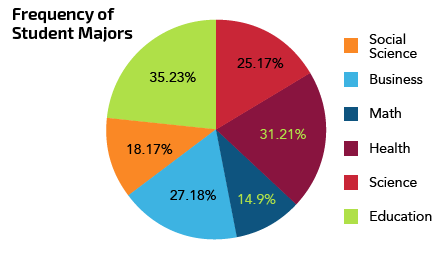Figure 2.8: Pie chart of student majors​

### Ogives

An ogive can be used to show how many data values lie below (or above) a particular value in a data set. The example below shows cumulative frequencies; ogives can also be constructed to show cumulative percent by changing the values on the vertical axis.

To construct an ogive:

Step 1: Construct a frequency table with appropriate classes.

Step 2: Draw the axes of the graph with an x (horizontal) axis and a y (vertical) axis.

Step 3: Enter the lower class limit of the first class along the horizontal x-axis.

Step 4: Enter the upper class limits of each class along the horizontal x-axis.

Step 5: Enter the cumulative frequencies on the vertical y-axis.

Step 6: Plot the cumulative frequencies above their respective class limits.

Step 7: Connect the plotted points by drawing a freehand curve through them.

Example: Ogive

The ogive example below shows the cumulative frequencies of the placement test scores from a previous example. Note how the line begins at zero frequency at the beginning of the first class and indicates the cumulative frequency values for each succeeding class, up to the total of all 25 scores.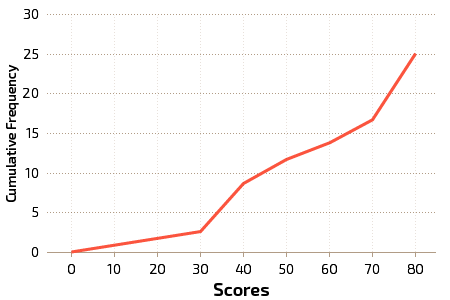Figure 2.9:​ Ogive Showing Placement Test Scores

### Stem and Leaf Plots

A more recent addition to the statistician’s analytical bag of tricks is the stem and leaf plot, developed by John Tukey in 1977. It provides much the same information as a histogram but has the advantage of retaining the original data values. It is also useful as a convenient way to sort data.

A stem and leaf plot is really a special kind of table where each data value is split into two parts. The first digit (or maybe two digits) becomes the “stem” and the last digit becomes the “leaf,” with a vertical line separating them. So the number 43 might end up as 4 | 3, with 4 as the stem and 3 as the leaf. You can see more in the example below.

To construct a stem and leaf plot the leaves should be single digits, while the stem provides the “base” that completes each value.

To construct a stem and leaf plot:

Step 1: List the stems to the left of a vertical line (use the | key on your keyboard).

Step 2: For each data entry, list a corresponding leaf to the right of its stem.

Step 3: To create an ordered stem and leaf plot, arrange the leaves in order from lowest to highest.

Step 4: Create a key that serves as an example to interpret the scores in the plot.

Example: Stem and Leaf Plot

The stem and leaf plot below summarizes the placement test scores displayed previously. As a refresher, the raw scores were 12, 72, 26, 77, 38, 34, 55, 67, 64, 51, 43, 48, 69, 77, 73, 44, 32, 8, 36, 78, 74, 33, 77, 72, 32. It is easy to see from the graph how the frequencies vary and to identify the high and low points in the distribution. The key provides the “solution” to interpreting and reconstructing the scores.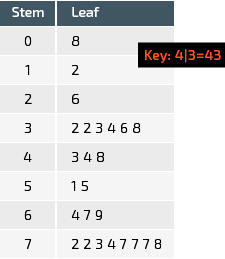Figure 2.10: Stem and leaf plot​

### Dot Plots

Another way to present quantitative data is by using a dot plot. The dot plot involves plotting each data point above a horizontal axis where individual values or classes are displayed. It provides a quick visual reference to the spread of the data and points out unusual data values like outliers.

To construct a dot plot:

Step 1: Draw the horizontal axis and label with individual data values or classes.

Step 2: Plot points above each value or class so that each value in the data set is represented.

Step 3: If duplicate values exist, plot a point for each instance of the value.

Example: Dot Plot

The dot plot below summarizes the placement test scores from the previous example. Note how it provides an easy to grasp visual “take” of the data and makes it easy to see the spread of the data.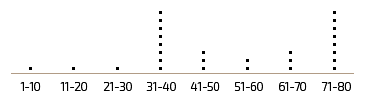Figure 2.11: Dot plot​

### Numbers Don’t Lie . . .

A bad chart choice can make it very difficult for your readers to understand just what it is all those numbers really mean. Before you settle on a chart type to use in summarizing and presenting your data, think about what chart type will work best to get your point across. Try not to rely on verbal or written explanations to interpret the chart’s meaning for your audience, but try to ensure the chart will “speak” for itself.

Understanding statistics is becoming a large part of media literacy. Often times, statistics posted in the news can be misleading. Here's a good example to hopefully make you more critical of statistics we see in everyday life:

2.07

Match the chart types with a characteristic or function from the list.

Premise
Response
1

Histogram

A

Shows frequencies of categories

2

Bar Chart

B

Visually shows proportions

3

Pie Chart

C

Key provides “solution”

4

Ogive

D

Quick visual reference of spread of data

5

Stem and Leaf Plot

E

Shows frequencies of numerical data

6

Dot Plot

F

Shows cumulative frequency

## Modality of a Distribution

The modality of a distribution can take several forms. It provides information about the general shape of a distribution and how scores within the distribution trend, along with how scores are concentrated around points or classes in the distribution.

The mode of a distribution of scores is defined as the score that occurs the most often. It also represents the highest peak or column in a graphed frequency distribution.

A unimodal distribution of scores is described as having one highest frequency, or value.

A bimodal distribution is one with two equal or approximately equally high score frequencies. Note that a distribution with two distinct peaks is not considered bimodal unless the peaks are very nearly equal.

A multimodal distribution is one with multiple, approximately equal peaks. A bimodal distribution could be considered as a special case of a multimodal distribution.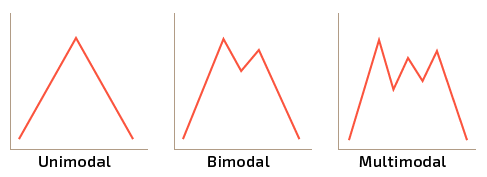Figure 2.12: Types of modality​

## Skewness

The presence or absence of skew in a distribution tells us more about how scores are dispersed within that distribution.

A skewed distribution has many more scores on one side of a graph of the distribution than on the other side. This is an indication of the skewness of the data set. This is frequently a result of having outliers in the data, i.e., data values that have the effect of extending the tail of the distribution in one direction or the other.Figure 2.13: Skewed bar chart​

Consider the distribution in Figure 2.13, the bars on the right side of the distribution taper differently than the bars on the left side. These tapering sides represent the tails, and they provide a visual means for determining which of the two kinds of skewness a distribution may have: a tail extending to the right is an indication of positive skew, while a tail extending to the left indicates negative skew.

### Negative and Positive Skew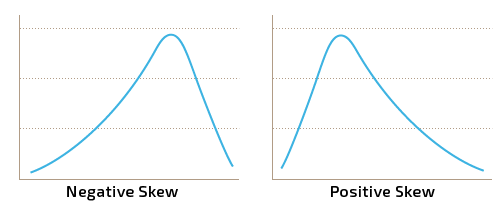Figure 2.14: Negative vs. positive skew​

Negative skew: The left tail is longer; the mass of the distribution is concentrated on the right of the figure. It has relatively few low values. The distribution is said to be left-skewed or negatively skewed. The graph to the left, above, is an illustration of negative skew.

Positive skew: The right tail is longer; the mass of the distribution is concentrated on the left of the figure. It has relatively few high values. The distribution is right-skewed or positively skewed. The graph to the right, above, is an illustration of positive skew.

Skewness can also be a result of either a floor effect or a ceiling effect.

•  A floor effect occurs when scores pile up against some lower limit, resulting in a positive skew, for example, as in the case of an exam that is too difficult for the target population.
•  A ceiling effect occurs when scores pile up against some upper limit, resulting in a negative skew, for example, as in the case of an exam that is too easy so that most testers score very high.

2.08

The modal score is approximately _____.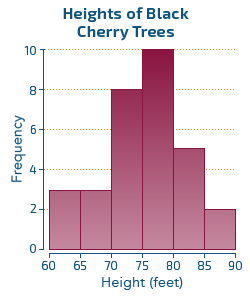A

74

B

70

C

77

D

80

2.09

This chart is an example of a _______.A

Category chart

B

Histogram

C

Summary chart

D

Bar chart

2.10

Does this distribution show skew?A

No, it is perfectly symmetrical

B

Yes, it has negative skew.

C

No, it is unimodal.

D

Yes, it is positively skewed.

## Measures of Central Tendency

Statistical analysis of data often begins by determining where the middle, or center, of the distribution lies. It turns out that there are no less than three commonly accepted measures of central tendency. Each is useful to know, and each provides important information about the distribution.

Mean: The mean of a set of scores is the sum of the scores divided by the number of scores. It is sometimes called the arithmetic mean, or average. There are two ways to represent the mean.

•  If the entire population of scores is known, the population mean is represented by μ (mu, aka lower case Greek M). It is calculated as:

where N represents the number of scores in the population.

•  If the scores are a subset of scores, as in a sample, the sample mean is represented by x̅ (x-bar). It is calculated as:

where n represents the number of scores in the sample.

The mean is the “most used” measure of central tendency for statistical purposes. It is used in many of the formulas and calculations you’ll be using in this course.

Median: The median is the midpoint score. If all the scores are arranged in a row, and there is an odd number of scores, it is the middle score. If there is an even number of scores, it is the value midway between the two in the middle. It is used to determine if a score is in the upper or lower half of a distribution.

Mode: The mode is the most frequently appearing score. A distribution may be bimodal or multi-modal if there are two or more obvious “peaks.”Figure 2.15: Mean, median and mode​

In the example above, notice how the median and mean are “pulled” to one side of the peak in the direction of the skew. Both are affected by extreme values in the distribution; the mean is the most sensitive to outliers, or extreme scores, in a distribution.

You can see for yourself how this works by selecting and toggling data points in the demonstration below.This Content is Locked
Only a limited preview of this text is available. You'll need to sign up to Top Hat, and be a verified professor to have full access to view and teach with the content.

Example: Find the Mean, Median and Mode of a Data Set

Assume a researcher is conducting research on the response time (RT) of participants in her study to a visual stimulus that is presented at random intervals. The task is to click a mouse when the target stimulus appears on a computer screen. The RT is measured in milliseconds. She collected data from a sample of 16 participants.

The data set is 34, 68, 54, 48, 46, 55, 74, 42, 62, 66, 71, 39, 44, 54, 58, 47.

The researcher then calculated the mean, median and mode as follows:

The mean

The median is the average of the two middle scores since there is an even number of scores in the distribution:

34 39 42 44 46 47 48 54 54 55 58 62 66 68 71 74

The median = (54 +54)/2 = 108/2 = 54

The mode is the most frequently appearing score = 54.

### Determine the Mean of a Grouped Data Set

The mean can also be approximated from a grouped frequency table. This is sometimes a good alternative when there is a large number of scores and it is useful to group the data in a grouped frequency table. The procedure is as follows:

Step 1: Find the midpoint of each class.

Step 2: Find the sum of the products of the midpoints and the class frequencies.

Step 3: Find the sum of the frequencies.

Step 4: Find the mean of the frequency distribution.

Example: Find the Mean of a Grouped Frequency Table

Assume the scores from the RT experiment above were grouped into a frequency table as below, and the researcher then wanted to calculate the mean of the grouped data. The data set was 34 39 42 44 46 47 48 54 54 55 58 62 66 68 71 74.Figure 2.16: RT scores​

The mean is calculated as:

Note how the mean, as calculated from the grouped data, varies slightly from the mean as calculated from the raw ungrouped data. Some information is lost when data is grouped, but the grouped data nevertheless offer a good approximation of measures.

2.11

Use the following data set to answer the question: 63, 89, 92, 73, 79, 72, 34, 36, 94, 21, 25, 93, 22, 90, 79

Calculate the mean of the data set.

2.12

Use the following data set to answer the question: 63, 89, 92, 73, 79, 72, 34, 36, 94, 21, 25, 93, 22, 90, 79

Calculate the median of the data set.

2.13

Use the following data set to answer the question: 63, 89, 92, 73, 79, 72, 34, 36, 94, 21, 25, 93, 22, 90, 79

What is the mode of the data set?

2.14

What do your answers to the previous three questions tell you about the distribution?

A

Nothing much

B

It is positively skewed

C

It is multimodal and negatively skewed

D

It is unimodal and negatively skewed

## Quartiles, Deciles, and Percentiles

Another way to specify position in a data set is to use fractiles. These numbers can be used to divide an ordered data set into equal parts.Figure 2.17: Fractile definitions & symbols​

### Interpretation

Fractiles, especially percentiles, are frequently used by psychologists and health professionals to describe characteristics of an individual in relation to a distribution of characteristics or scores.

•  A student’s score on a standardized test might be reported as “at the 60th percentile,” meaning that the student scored as high or higher than 60% of all students taking that test.
•  A child’s height might be reported as “above the third quartile,” meaning that the child’s height is in the upper 25% of all children of that particular group.

### Calculating Fractiles

Quartiles divide a data set into four equal parts. The second quartile, Q2, is equal to the median of the data set. The median is used instead of the mean because the median is the center point, or middle score, of the distribution. The mean is theoretical and may or may not appear as an actual score.

Q1 and Q3 are the 25th and 75th percentiles, respectively. Q2 is also the median, equal to the 50th percentile.

Deciles divide the data into tenths. The 1st decile, D1, is equal to the 10th percentile, etc.

Percentiles divide the data into hundredths. It is the value below which a percentage of the data lies.

Quartiles

To determine quartiles, start by locating the median of the data. That is Q2 and it divides the data set into two equal halves. You can find Q1 and Q3 by locating the medians of the lower and upper halves of the data, respectively. Use the same procedure as for any other median.

Deciles

Use the procedure for finding percentiles, just below, to locate D1, D2 . . . D9.

Percentiles

There are several commonly accepted methods for finding percentiles, all of which give slightly different results. For simplicity, we can use a basic formula that will approximate a given percentile of a set of scores, summarized in the table below:Figure 2.18: Calculating percentiles​

2.15

Ten students took a placement test to see if they could bypass a prerequisite requirement for a course. Out of 100 points possible, the scores were: 45, 62, 63, 58, 81, 77, 64, 69, 82, 51.

The student who scored 69 was at what percentile?

2.16

Ten students took a placement test to see if they could bypass a prerequisite requirement for a course. Out of 100 points possible, the scores were: 45, 62, 63, 58, 81, 77, 64, 69, 82, 51.

What is Q$_2$ on this administration of the test?

2.17

Ten students took a placement test to see if they could bypass a prerequisite requirement for a course. Out of 100 points possible, the scores were: 45, 62, 63, 58, 81, 77, 64, 69, 82, 51.

What were the scores of the students who scored above the third quartile? Separate your answer with a comma and a space.

## Dispersion of Scores (Variability)

It is important to note that measures of central tendency only locate the center of a distribution. To completely define the distribution, we also need to know how the scores are dispersed about that center – i.e., we also need to know the variability. The two distributions below may have the same mean, but they are very different in terms of their dispersion!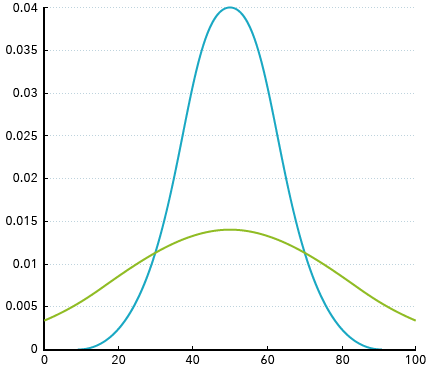Figure 2:19: Dispersion of data​

### Measures of Variability

The two most-used measures of dispersion, or variability, are the variance and the standard deviation. The concept of deviation is key to an understanding of these measures.

### Deviation

Deviation is the distance of a score x from the mean of the distribution it is included in.

•  If the entire population of scores is given, deviation is represented as (x – μ), the distance between a raw score x and the population mean, mu.
•  If the distribution is a subset of scores, as in a sample, it is represented as (x – x̅), the distance between a raw score x and the sample mean, x-bar.

Determining the deviation in a set of scores is the first step in calculating the variance and standard deviation associated with those scores.

•  A sum of deviations is the sum of the deviations of the individual scores: ∑ (x - μ) or        ∑ (x – x̅), depending on whether the distribution is a population or a sample.

Note: The sum of deviations from the mean in a distribution always equals zero. Since the mean is the average of the scores in the distribution, the differences between the mean and scores above the mean will be offset by the differences below the mean.

•  A squared deviation is the deviation of a score, squared: (x – μ)2
•  A sum of squared deviations, aka a sum of squares, is the sum of the squared deviations from the mean: ∑ (x – μ)2.

Example: Calculate Deviation and Sum of Squared Deviations

Given a population of scores as 4, 6, 3, 5, the table below shows how to figure the deviation of each score from the mean, the squared deviation, and the sum of squared deviations, or “sum of squares,” at the bottom, right. Using a table such as this greatly simplifies the operations and makes it easy to keep track of the values as you go.

Step 1: Enter the scores in the Score column.

Step 2: Sum that column and divide by the number of scores (N) to calculate the mean of the scores.

Step 3: Enter the mean next to each score in the set.

Step 4: Subtract the mean from each score and enter that difference in the Deviation column.

Step 5: Square each deviation and enter that result in the Squared Deviation column.

Step 6: Sum that column to get the sum of squared deviations.Figure 2.20: ​Deviations from the Mean​

### Parameters

A parameter is a measure of a population characteristic. By convention, the notation is based on Greek letters such as µ (mu), σ (sigma), ρ (rho), etc.  See this guide to statistical notation for review and reference.

### Statistics

A statistic is a measure of a sample characteristic. The notation is based on Roman letters such as x, s, p, r, etc. See this guide to statistical notation for review and reference.

Note: Individual data values, or “scores,” are represented by x’s in both population and sample formulas.

## Population Variance and Standard Deviation

The variance is defined as the average of the squared deviations from the mean. If the values for the entire population are known, the population variance is calculated using N to represent the total number of scores in the population.

There are two reasons for squaring the deviations used in calculating the variance:

•  Squaring “weights” the value in favor of the larger deviations, augmenting the effect of “outliers” in the distribution.
•  Squaring makes all the values positive.

The population variance is represented by a lower case Greek sigma squared, σ2, and is calculated as:

To calculate the population variance for the example above, divide the sum of squares by N:

### Population Standard Deviation

The standard deviation is defined as the positive square root of the variance. Taking the square root returns the value to the original scale of measurement so that it is expressed in units of the original data set.

Since it is expressed in units of the original data set, the standard deviation summarizes variability in a distribution in a form that is specific to that particular distribution. It also serves as a locator for scores in the distribution, in that once we know the standard deviation, we can determine how many standard deviations a given raw score is from the mean. As we’ll see in the next chapter, that can be important information.

The population standard deviation is represented by a lower case Greek sigma, σ, and is calculated as:

To calculate the population standard deviation for the example above, take the positive square root of the variance:

## Sample Variance and Standard Deviation

If all of the scores in the population are not known, as is the case when working with a subset of scores like a sample, the variance can be estimated from the scores that are known. In this case, n is used to represent the number of scores in the sample and the calculation changes slightly. Instead of dividing by n, the total number of scores in the sample, the sum of squared deviations is divided by n-1.

Note: When a sample variance is calculated, the sum of the scores is divided by n – 1 instead of n. This is done to correct for the tendency of the sample variance to slightly underestimate the population variance. This correction will be important in future lessons when using the sample mean as an estimate of the population parameter.

The sample variance is represented by s2 and is calculated as:

If the previous data set represented a sample instead of a population, its variance would be calculated as:

### Sample Standard Deviation

The sample standard deviation is the positive square root of the variance:

To calculate the population standard deviation for the example above, take the positive square root of the variance:

### How Is This Useful?

It turns out that knowing the standard deviation associated with a measure can be extremely useful. As we’ve seen, it is a measure of the variability within a distribution, but beyond that, it can also provide information about extreme data points that may appear and the probabilities associated with those. For example, a data point that is several standard deviations from the mean may be just due to random variation, but it could also signify an unusual event that needs to be investigated, as the video here points out.

For an interesting example of how variance might be used to solve a problem, check out this video about Shakespeare's plays.

2.18

You previously found the mean of this data set. Use that in answering the question. 63, 89, 92, 73, 79, 72, 34, 36, 94, 21, 25, 93, 22, 90, 79

Calculate the sum of squares of the data set.

2.19

You previously found the mean of this data set. Use that in answering the question. 63, 89, 92, 73, 79, 72, 34, 36, 94, 21, 25, 93, 22, 90, 79

Calculate the sample variance of the data set.

2.20

You previously found the mean of this data set. Use that in answering the question. 63, 89, 92, 73, 79, 72, 34, 36, 94, 21, 25, 93, 22, 90, 79

Calculate the sample standard deviation of the data set.

### Question 2.21

2.21

When calculating a standard deviation, what is the point of squaring and then un-squaring the data?

Click here to see the answer to Question 2.21.

### Question 2.22

2.22

Calculate the population standard deviation of the hypothetical data set just above and compare it to the standard deviation you calculated in Question 2.20. What do you see? Why do you think it might be important that the results be the “way they are?”

Click here to see the answer to Question 2.22.

## Rounding Rules

When calculating means, variances, and standard deviations, rounding should be the last step in the process. It should not be done until the final answer is calculated.

The mean should be rounded to one more decimal place than the original data set.

The standard deviation should also be rounded to one more decimal place than the original data. The variance should not be rounded unless it is the final answer to a question.

Example: Rounding Values

Given a data set as 6, 8, 17, 4, 21, 8, 12, 9. 16, 19, 17, 11, 13, calculate the mean and standard deviation. Using a technology tool for the calculations, the results are given as:

Note that the results are expressed in tenths, one more decimal place than the original data set.

There is an online standard deviation calculator here. It is especially useful for checking answers so that you can see if you’re on the right track with calculations.

## Case Study: Statistics Aren't the Whole Story

Presenting data using charts and graphs is a very useful method of summarizing the data. It is important to bear in mind, though, that as consumers of statistics we all need to be aware of possible inappropriate uses of statistics. Part of your takeaway from this text, in fact, will be a heightened awareness of uses and abuses of statistics and the importance of presenting data in ways that do not lead to misrepresentation, intentional or not. Watch the following video, How Statistics Can Lie, to see more.

Watch below to see another example of how statistics can be manipulated.

Finally, read through this case study from the American Association for the Advancement of Science that takes a look at sex bias in graduate admissions.

### Case Study Question 2.01

Case Study Question 2.01

What did the narrator in the “How Statistics Can Lie” video mean by, “Statistics do not create themselves; people create statistics,” and why is that important?

Click here to see the answer to Case Study Question 2.01.

### Case Study Question 2.02

Case Study Question 2.02

The narrators in the two videos comment that people often take statistics as absolute truth. Why do you think people are prone to do that? What kinds of problems does that leave us open to?

Click here to see the answer to Case Study Question 2.02.

### Case Study Question 2.03

Case Study Question 2.03

Describe one other “take away” from the videos.

Click here to see the answer to Case Study Question 2.03.

### Case Study Question 2.04

Case Study Question 2.04

Describe an example of a misleading statistic that you’ve heard as part of a marketing campaign.

Click here to see the answer to Case Study Question 2.04.

### Case Study Question 2.05

Case Study Question 2.05

In the summary of the article on admissions bias, the authors conclude that the results show “a clear but misleading pattern of bias.” What is your interpretation of that?

Click here to see the answer to Case Study Question 2.05.

### References

P. J. Bickel, E. A. (1975, Feb 7). Sex Bias in Graduate Admissions: Data from Berkeley. Retrieved from American Association for the Advancement of Science: http://www.unc.edu/~nielsen/soci708/cdocs/Berkeley_admissions_bias.pdf

## Pre-Class Discussion Questions

### Class Discussion 2.01

Class Discussion 2.01

What purpose do descriptive statistics serve?

Click here to see the answer to Class Discussion 2.01.

### Class Discussion 2.02

Class Discussion 2.02

Describe the organization of a frequency table.

Click here to see the answer to Class Discussion 2.02.

### Class Discussion 2.03

Class Discussion 2.03

Compare histograms and bar charts.

Click here to see the answer to Class Discussion 2.03.

### Class Discussion 2.04

Class Discussion 2.04

Differentiate the mean, median and mode of a data set.

Click here to see the answer to Class Discussion 2.04.

### Class Discussion 2.05

Class Discussion 2.05

What important information does the standard deviation convey?

Click here to see the answer to Class Discussion 2.05.This Content is Locked
Only a limited preview of this text is available. You'll need to sign up to Top Hat, and be a verified professor to have full access to view and teach with the content.

## Answers to In-Chapter Questions

### Answer to Question 2.21

It first makes all values positive so they are in effect absolute values and weights extreme values. It then returns the results to the scale of the original data so that direct comparisons can be made.

Click here to return to Question 2.21.

### Answer to Question 2.22

The population value is slightly less than the sample value. In drawing statistical conclusions about the data when using the sample statistic as an estimate of the population parameter, underestimating the variability of the data could lead to incorrect conclusions. Slightly overestimating the variability is a more conservative approach.

Click here to return to Question 2.22.

## Answers to Case Study Questions

### Answer to Case Study Question 2.01

It can be tempting to accept statistics at “face” value, without bothering to question their origin or production. After all, they are “just numbers,” right? But keep in mind that these numbers go through some manipulations in order to be presented, and that can open the door to “tweaking” of the data, either intentionally or unintentionally.

Click here to return to Case Study Question 2.01.

### Answer to Case Study Question 2.02

Statistics are often presented by presumed “authority figures.” That may lead the audience to accept the information and any inferences being made as having validity, whether or not that confidence is really justified. This may create issues when false or erroneous information is accepted at face value and mislead people into believing false or unjustified conclusions.

Click here to return to Case Study Question 2.02.

### Answer to Case Study Question 2.03

Use common sense when making decisions about the reliability of claims and statistics! If it doesn’t pass the “sniff test,” then be wary.

Click here to return to Case Study Question 2.03.

### Answer to Case Study Question 2.04

Anyone remember Colgate’s claim that 80% of dentists recommended the brand? You won’t be seeing that slogan again, at least not in the UK. Consumers were led to believe that 80% of dentists recommended Colgate while 20% recommended other brands. It turns out that when dentists were surveyed, they could choose several brands — not just one. So other brands could be just as popular as Colgate. This completely misleading statistic was banned by the Advertising Standards Authority.

Click here to return to Case Study Question 2.04.

### Answer to Case Study Question 2.05

This echoes the point made in the previous videos as well. Depending on how the data are managed and interpreted, conflicting conclusions are often not only possible but often inevitable.

Click here to return to Case Study Question 2.05.

## Answers to Pre-Class Discussion Questions

### Answer to Class Discussion 2.01

Descriptive statistics provide a means of organizing and summarizing data so that essential characteristics can be easily grasped.

Click here to return to Class Discussion 2.01.

### Answer to Class Discussion 2.02

Frequency tables are used to organize data into classes and frequencies within each class. This provides an easy to follow summary of the dispersion of the data.

Click here to return to Class Discussion 2.02.

### Answer to Class Discussion 2.03

Histograms are organized as contiguous columns, summarizing frequencies of continuous numerical data that has been organized into classes.

Bar charts are similar, but the columns are not contiguous, representing frequencies of nominal data, or categories.

Click here to return to Class Discussion 2.03.

### Answer to Class Discussion 2.04

The mean is the arithmetic average of the data set, calculated as ∑x / n.

The median is the midpoint of the data set; half the scores are above and half are below the median.

The mode is the most frequently occurring score. A data set may have one or more modes so it may be termed unimodal, bimodal, or multimodal.

Click here to return to Class Discussion 2.04.

### Answer to Class Discussion 2.05

The standard deviation is a measure of variability within a distribution. The larger the standard deviation, the more spread out the data are. It also serves as a “locator” for scores, in that individual scores can be compared in terms of their distance from the mean of the distribution, expressed in standard deviations.

Click here to return to Class Discussion 2.05.

### Image Credits

 Image courtesy of Ankara'dan in the Public Domain.